# Conjoint Analysis - Calculations / Methodology

Conjoint analysis is a survey based statistical technique used in market research that helps determine how people value different attributes (feature, function, benefits) that make up an individual product or service.

The objective of conjoint analysis is to determine what combination of a limited number of attributes is most influential on respondent choice or decision making. A controlled set of potential products or services is shown to survey respondents and by analyzing how they make preferences between these products, the implicit valuation of the individual elements making up the product or service can be determined. These implicit valuations (utilities or part-worths) can be used to create market models that estimate market share, revenue and even profitability of new designs.

## Conjoint Analysis – Attribute Importance

To view detailed conjoint analysis

Go to: Login » Surveys » Reports » Choice Modelling » Conjoint AnalysisCalculating Attribute Importance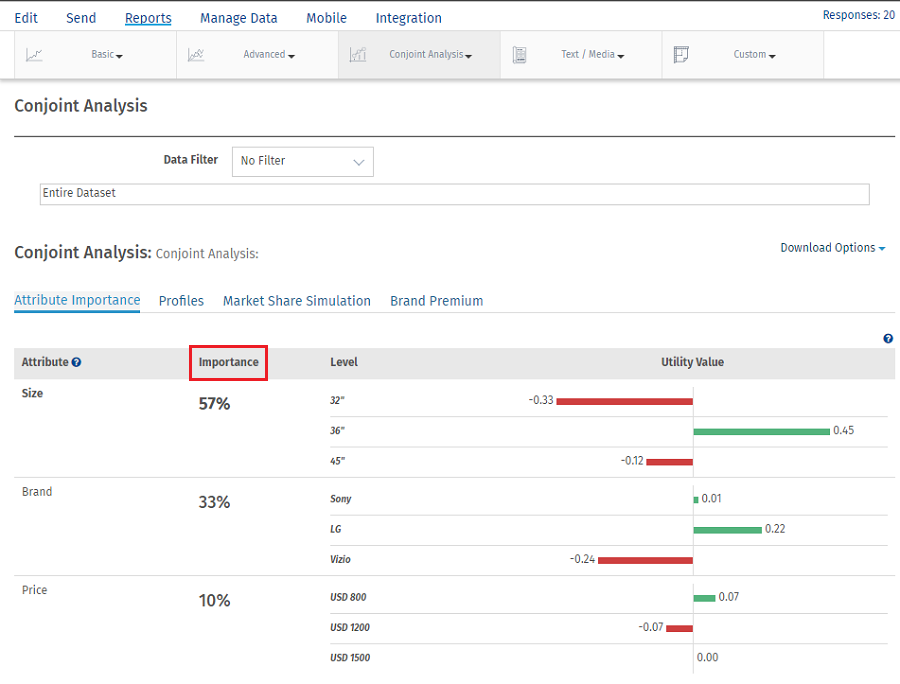Sometimes we want to characterize the relative importance of each attribute. We can do this by considering how much difference each attribute could make in the total utility of a product. That difference is the range in the attribute’s utility values.

Importance measures are ratio-scaled,but they are also relative,study-speciﬁc measures. An attribute with an importance of twenty percent is twice as important as an attribute with an importance of ten, given the set of attributes and levels used in the study. That is to say, importance has a meaningful zero point,as do all percentages. But when we compute an attribute’s importance,it is always relative to the other attributes being used in the study. And we can compare one attribute to another in terms of importance within a conjoint study but not across studies featuring different attribute lists.

Example:

Step 1: Calculate Attribute Utility Range

Utility Range = Highest Utility Value of an attribute - Lowest Utility Value of an attribute

For Price Range is calculated as 0.45-(-0.33) = 0.78
For Size Range is calculated as 0.22-(-0.24) = 0.46
For Brand Rage is calculated as 0.07-(-0.07) = 0.14

Step 2 : Calculate Total Attribute Utility Range

Total Utility Range = ∑ Utility Range

Total Attribute Utility Range = 0.78+0.46+0.14 = 1.38

Step 3: Calculating Relative Importance of attributes

Relative Importance of attribute = (Attribute Utility Range/Total Attribute Utility Range)*100

Importance of Price = (0.78/1.38)*100 = 56.52% Rounded off to 57%
Importance of Size = (0.46/1.38)*100 = 33.33% Rounded off to 33%
Importance of Brand = (0.14/1.38)*100 =10.14% Rounded off to 10%

## Calculating Utility Value

Conjoint utilities or part-worths are scaled to an arbitrary additive constant within each attribute and are interval data. The arbitrary origin of the scaling within each attribute results from dummy coding in the design matrix. We could add a constant to the part-worths for all levels of an attribute or to all attribute levels in the study, and it would not change our interpretation of the ﬁndings. When using a speciﬁc kind of dummy coding called effects coding, utilities are scaled to sum to zero within each attribute.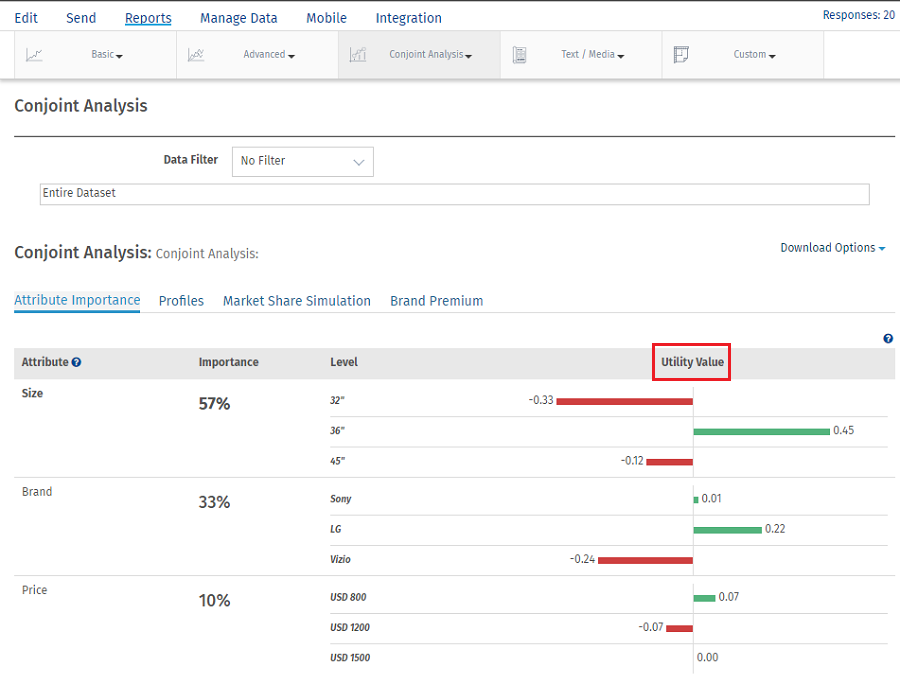Utility Value is calculated using part worth value. For every level of attribute summation of part worth is calculated. Then the average of the summation is taken to get the utility value of a particular level.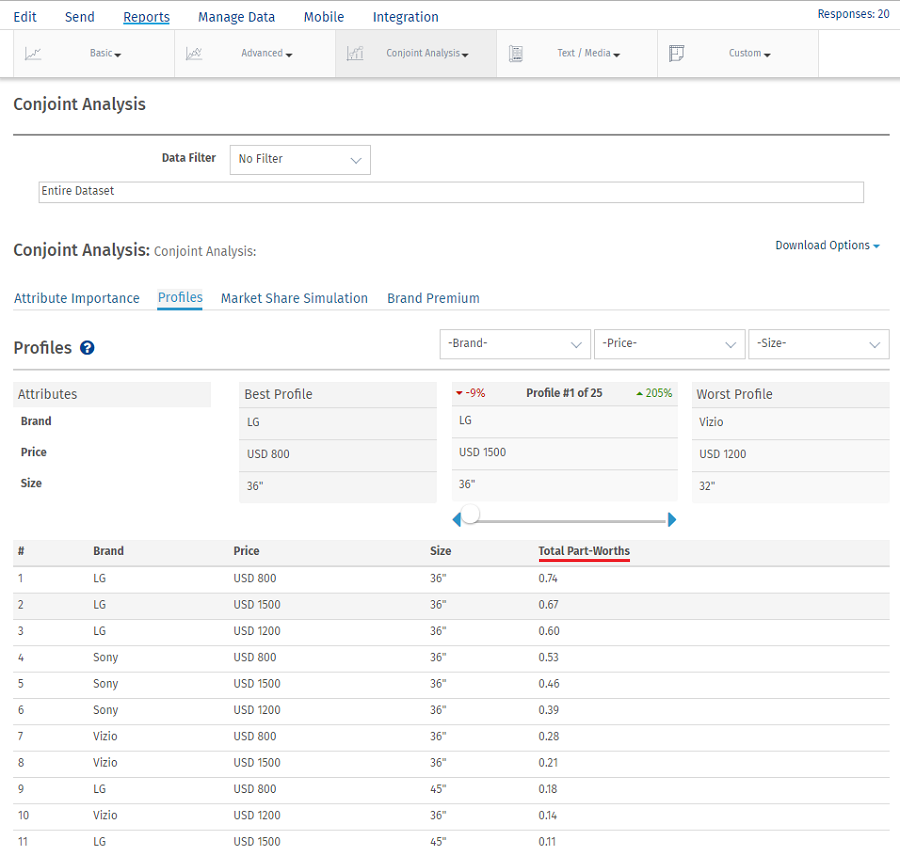Example: Utility value for size 36” is calculated by taking summation of Total part worths for 36” average of which will give utility value.

Summation of total part worths of 36” = 0.74+0.67+0.60+0.53+0.46+0.39+0.28+0.21+0.14 = 4.02

Average = 4.02/9 which gives utility value of 0.45 for attribute size with level 36’’

## Profiles

A conjoint profile is a set of attributes with different levels that are displayed for selection. Respondents would be shown a set of products, prototypes, mockups, or pictures created from a combination of levels from all or some of the constituent attributes and asked to choose from the products they are shown. Each example is similar enough that consumers will see them as close substitutes, but dissimilar enough that respondents can clearly determine a preference. Each example is composed of a unique combination of product features.

Under Conjoint Analysis, select the profile tab and you can view the best and worst profiles. The best profile is always displayed on the left and the worst profile on the right. In between, you can select a custom profile to see how it compares with the best and the worst profile.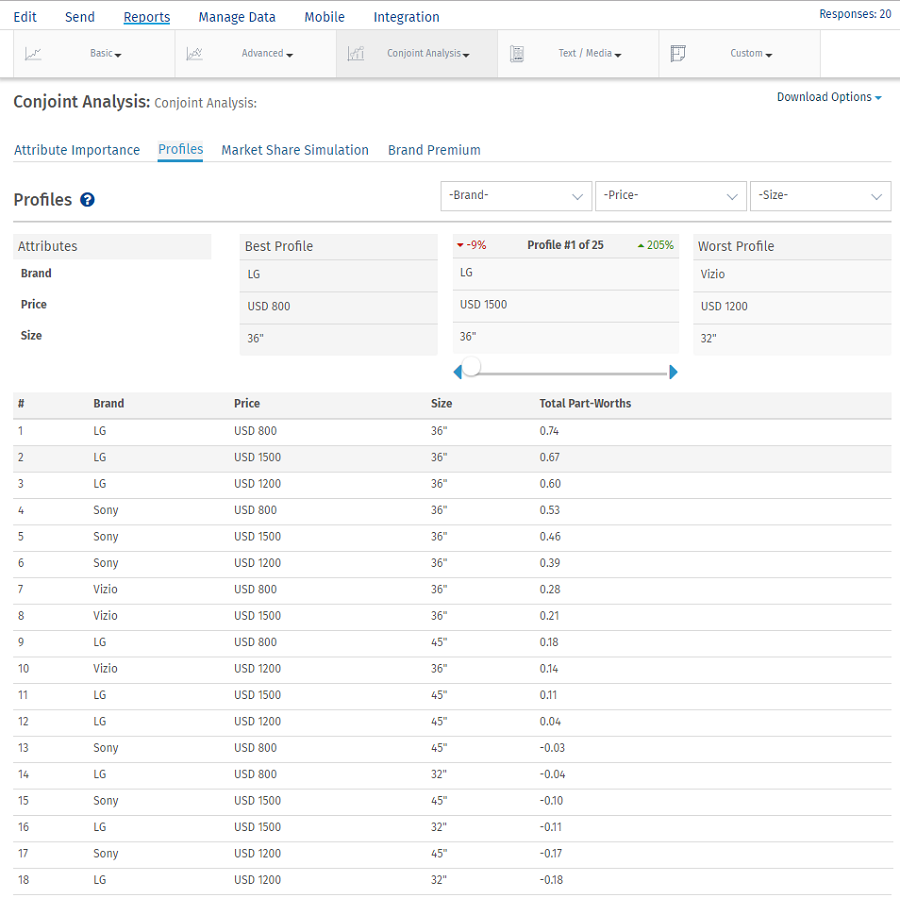For the selected profile, you can see the percentage point difference between the best profile and the selected profile in red.

The percentage point difference between the worst profile and the selected profile is displayed in green.

You can also limit/narrow down on the profiles by selecting specific levels for the attributes.

Attribute and level with highest Total Part Worth Value will be selected as the Best Profile and the one with lowest Total Part Worth value will be selected as the Worst Profile.

Calculating Part-Worths Values

We use the following algorithm to calculate CBC Conjoint Part-Worths:

Notation

Let there be R respondents, with individuals r = 1 ... R

Let each respondent see T tasks, with t = 1 ... T

Let each task t have C configurations (or concepts), with c = 1 ... C (C in our case is usually 3 or 4)

If we have A attributes, a = 1 to A, with each attribute having La levels, l = 1 to La, then the part-worth for a particular attribute/level is w’(a,l). It is this (jagged array) of part worths we are solving for in this exercise.

We can simplify this to a one-dimensional array w(s), where the elements are:{w’(1,1), w’(1,2) ... w’(1,L1), w’(2,1) ... w’(A,LA)} with w having S elements.

A specific configuration x can be represented as a one-dimensional array x(s), where x(s)=1 if the specific level/attribute is present, and 0 otherwise.

Let Xrtc represent the specific configuration of the cth configuration in the tth task for the rth respondent. Thus the experiment design is represented by the four dimensional matrix X with size RxTxCxS

If respondent r chooses configuration c in task t then let Yrtc=1; otherwise 0.

Utility Of A Specific Configuration

The Utility Ux of a specific configuration is the sum of the part-worths for those attribute/levels present in the configuration, i.e. it is the scalar product x.w

The Multinomial Logit Model

For a simple choice between two configurations, with utilities U1 and U2, the MNL model predicts that configuration 1 will be chosen

EXP(U1)/(EXP(U1) + EXP(U2)) of the time (a number between 0 and 1).

For a choice between N configurations, configuration 1 will be chosen

EXP(U1)/(EXP(U1) + EXP(U2) + ... + EXP(UN)) of the time.

Modeled Choice Probability

Let the choice probability (using MNL model) of choosing the cth configuration in the tth task for the rth respondent be:

Prtc=EXP(xrtc.w)/SUM(EXP(xrt1.w), EXP(xrt2.w), ... , EXP(xrtC.w))

Log-likelihood Measure

The Log-Likelihood measure LL is calculated as:Prtc is a function of the part-worth vector w, which is the set of part-worths we are solving for.

Solving For Part-worths Using Maximum Likelihood

We solve for the part-worth vector by finding the vector w that gives the maximum value for LL. Note that we are solving for S variables.

This is a multi-dimensional non-linear continuous maximization problem, and requires a standard solver library. We use the Nelder-Mead Simplex Algorithm.

The Log-Likelihood function should be implemented as a function LL(w, Y, X), and then optimized to find the vector w that gives us a maximum. The responses Y, and the design X are given, and constant for a specific optimization. Initial values for w can be set to the origin 0.

The final part-worths w are re-scaled so that the part-worths for any attribute have a mean of zero, simply by subtracting the mean of the part-worths for all levels of each attribute.

## Market Segmentation Simulator

The Conjoint Simulator is by far the most interesting aspect of Conjoint Analysis. This gives you the ability to "predict" the market share of new products and concepts that may not exist today. This also gives you the ability to measure the "Gain" or "Loss" in market share based on changes to existing products in the given market.

The first step in Conjoint Simulation is identifying and describing the different products or concepts that you want to investigate. We call these "Profiles". As mentioned earlier, any product or concept can be identified or created using the Attributes and Levels that have been defined for the study. For the purposes of the Conjoint Simulator, a product or concept comprises of all the Attributes and one level from each of the attributes.

For example one of the profiles could be: Brand - Sony, Price - \$899, Size - 42"

In most cases we would want to find out all the existing products that are available in that market segment and simulate the market share of the products to establish a baseline. Once this is established, it would be a good starting point for trying out new services and ideas and see how the market share shifts based on new products and configurations.

Go To : Login » Surveys » Reports » Choice Modelling » Conjoint Analysis

Click on Market Share Simulation.

Add profile as shown below and click on Run Simulation button. This provides the report with Vote Count and Market Share of respective concept.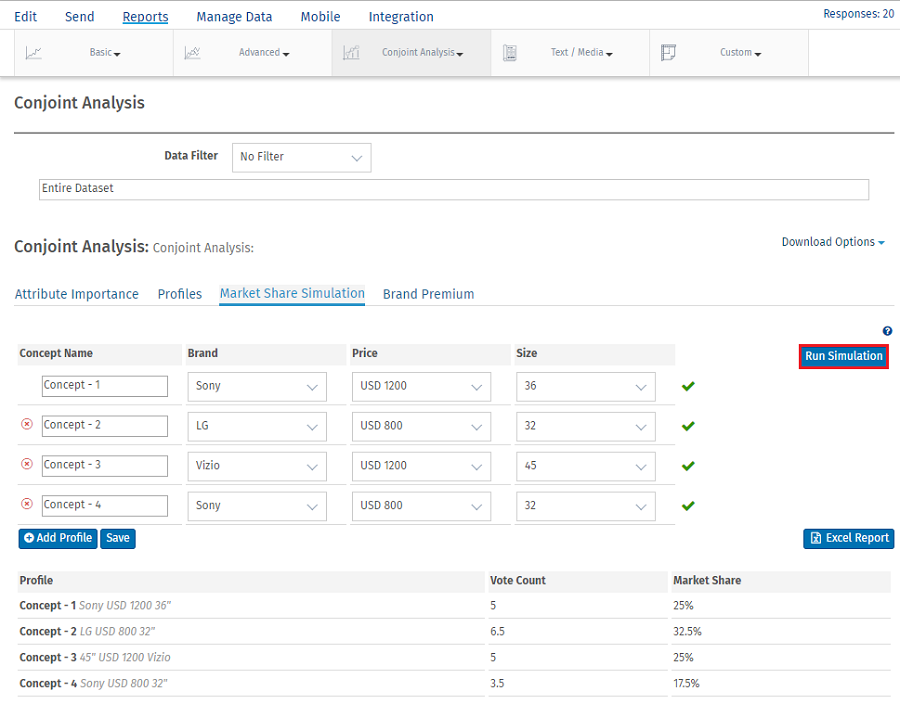Calculations for Vote Count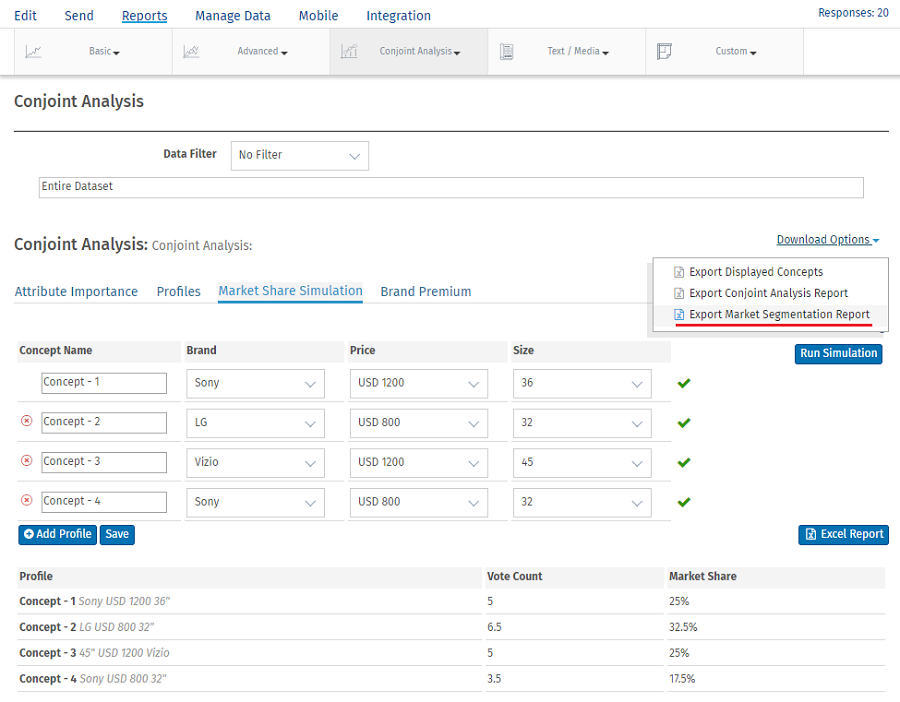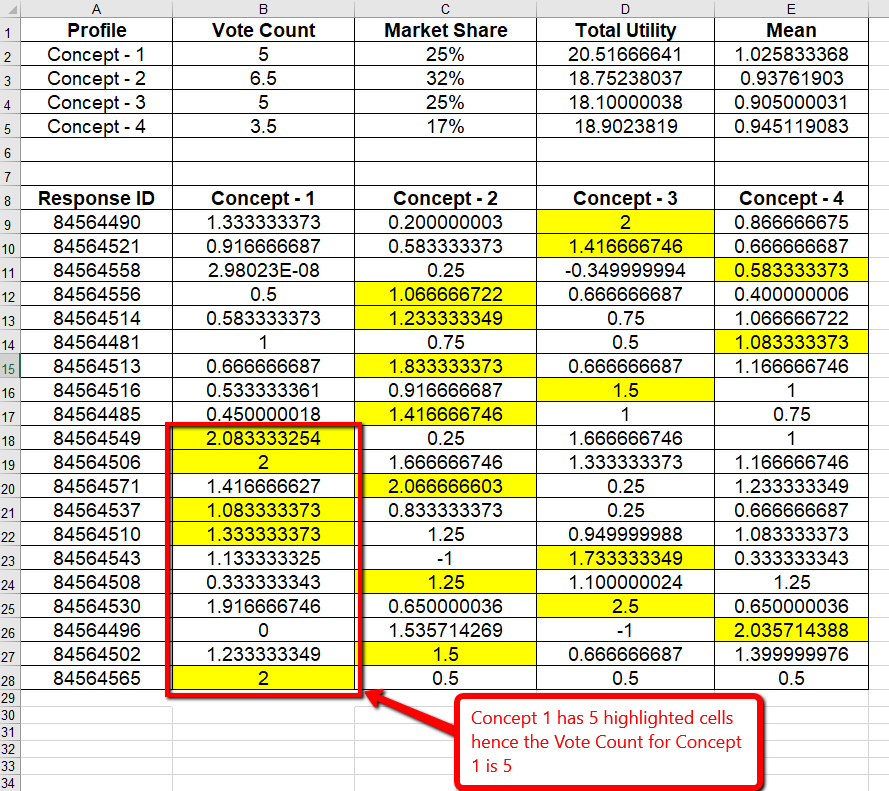Since in the example we added 4 concepts we have part worth value for 4 concepts.

Here the Utility value is derived from the following formula:

Utility Value = Σlog(e (W * Selected Configuration) / e (W * All Configurations))

• Where W is one-dimensional part-worth array.
If we have A attributes, a = 1 to A, with each attribute having La levels, l = 1 to La, then the part-worth for a particular attribute/level is w’(a,l). It is this (jagged array) of part-worths we are solving for in this exercise.
We can simplify this to a one-dimensional array w(s), where the elements are:{w’(1,1), w’(1,2) ... w’(1,L1), w’(2,1) ... w’(A,LA)} with w having S elements.
• Selected Configuration(levels selected in concept) is in form of array where selected= 1.
• All configuration(levels appeared in concept) is also in form of an array.
• We then take the dot product of (W * Selected Configuration) and (W * All Configurations) and impute in the mentioned formula to get the utility values.

We calculate the max of Utility value between 4 concepts row wise.In the above example max Utility value between 4 concepts are highlighted in yellow.

We then take the count of these highlighted cells which gives us vote count.If there is any tie then we take average.

In the above example, Concept 1 has 5 highlighted cells hence the Vote Count is 5.

Concept 2 has 7 highlighted values of which Response Id : 84564508 has two max values 1.25. In such tie situation we average out by ½ =0.5. (If 1.25 was present in one more concept than average would be ⅓ = 0.33) . Hence the Vote Count will be 6.5.

Likewise Vote Count is Calculated for Concept 3 and 4.

We take the Total of Vote Count = 5+6.5+5+3.5 = 20

Market Share = Individual Vote Count/Total Vote Count *100

Market Share of Concept 1 = (5/20)*100 = 25%
Market Share of Concept 2 = (6.5/20)*100 = 32.5%
Market Share of Concept 3 = (5/20)*100 = 25%
Market Share of Concept 4 = (3.5/20)*100 = 17.5%

## Price Elasticity

How much more will a customer pay for a Samsung versus an LG television? Assigning price as an attribute and tying that to an attribute returns a model for a \$ per utility distribution. This is leveraged to compute the actual dollar amount relative to any attribute. When the analysis is done relative to brand, you get to put a price on your brand.

What is Price Elasticity?

Price elasticity relates to the aggregate demand for a product and the shape of the demand curve. It is a characteristic of a product in a market. The way we calculate is plotting the demand (Frequency Count / Total Response) at different levels of price.

• Under Feature Type, select the Feature Type as Brand or Cost/Price.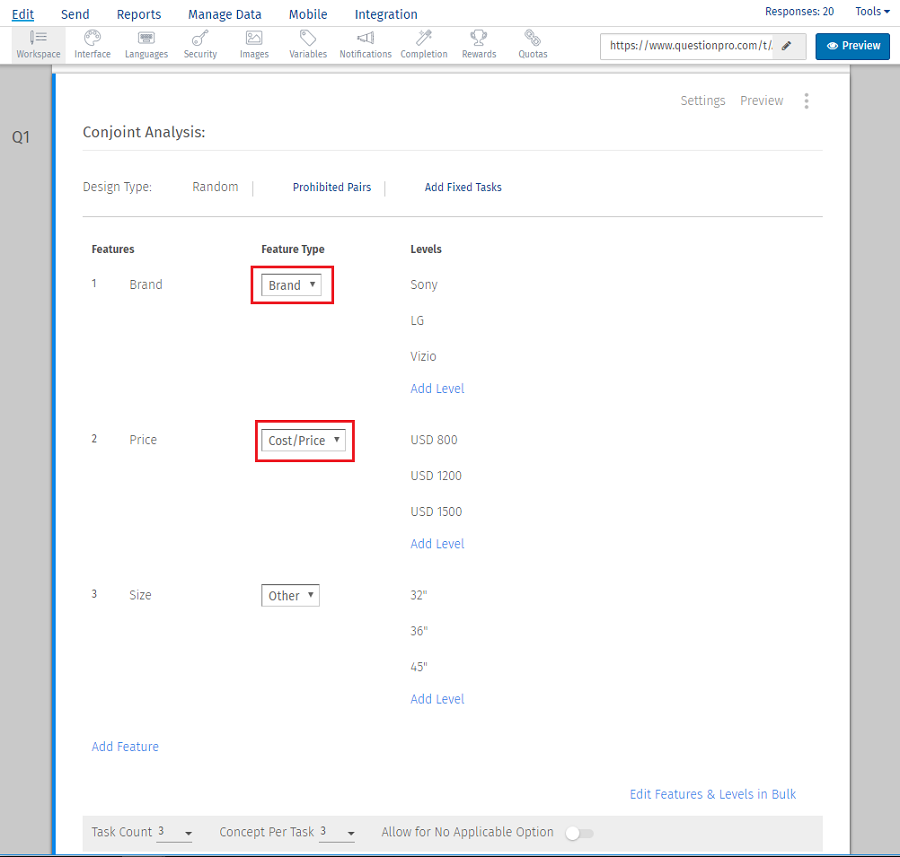Now you can check the Brand Premium under Login » Surveys » Reports » Choice Modelling » Conjoint Analysis » Brand Premium

Select Price Elasticity against, Brand or Size.

Price Elasticity Calculation

Example: Brand Sony at price \$800 gives 57%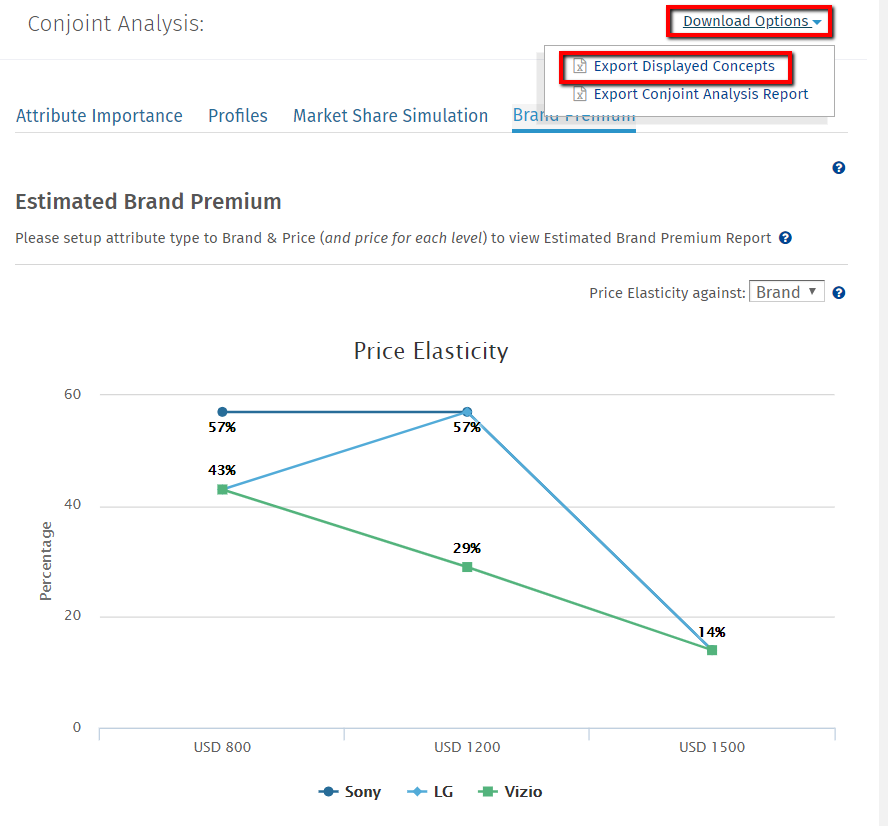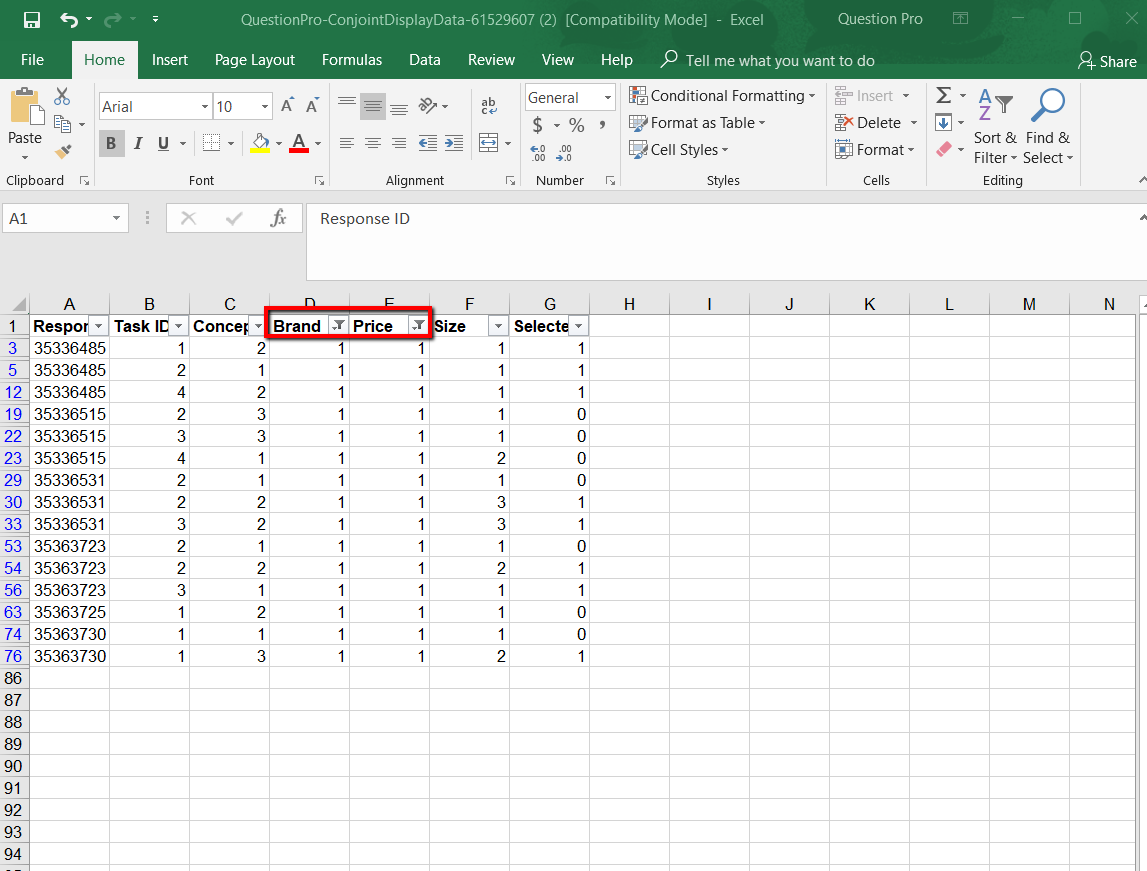For Brand = Sony (Numeric Coding = 1) and price = \$800 (Numeric Coding = 1)

Hence in report downloaded we filter price and brand with 1 and take the unique count of response ID with selected = 1. Overall we have 8 counts with selected = 1 of which actually there are 4 unique response ID(35336485,35336531,35363723,35363730).

Total responses collected = 7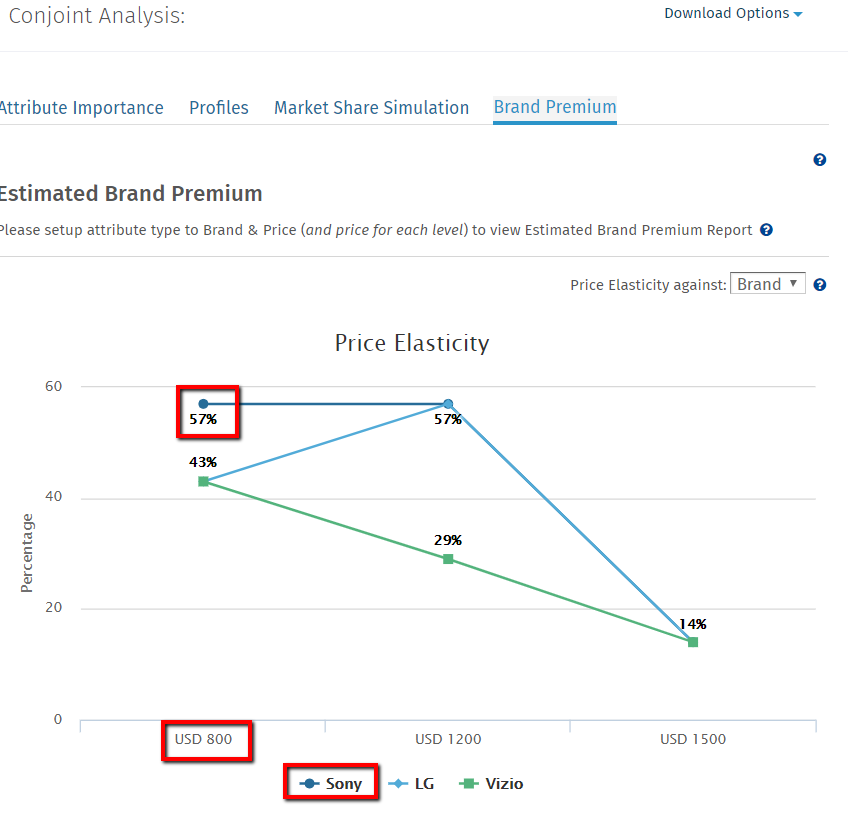Price Elasticity = Count of Unique Response ID for a particular feature level with selected is 1/Total Response Collected

Hence Price Elasticity for sony with price = \$800 is 4/7 = 57%